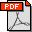Article Details

Analytical solution of the pseudo-3D model for hydraulic fracturing in a storage-dominated regime

Oleh   Egor V. Shel [-]
 Kontributor / Dosen Pembimbing : Grigory V. Paderin Jenis Koleksi : Jurnal elektronik Penerbit : Lain-lain Fakultas : Subjek : Applied physics Kata Kunci : Hydraulic fracture, P3D, Symmetric stress barriers, Equilibrium height growth, Geomechanics, Newtonian and non-Newtonian fluids Sumber : International Journal of Rock Mechanics and Mining Sciences 114 (2019) 92–100 Staf Input/Edit : Resti Andriani   Ena Sukmana File : 1 file Tanggal Input : 2019-01-11 13:27:122019_EJRNL_PP_EGOR_V_SHEL_1.pdf

Terbatas
» ITB

This paper deals with the so-called “pseudo-three-dimensional” (P3D) model of a hydraulic fracture with equilibrium height growth across two symmetric stress barriers. The key simplifying assumptions behind the P3D model are as follows: the fracture profile perpendicular to the main propagation direction is in a condition of plane strain; the equilibrium height and form are fully determined by the fluid pressure, rock stress contrast, and toughness; and in the equilibrium height growth P3D models, the pressure is uniform in each vertical crosssection. This particular model is assessed by first formulating the non-linear differential equations in the specific dimensionless form and finding an analytical solution in a storage-dominated regime. Whole differential equations were rewritten to correspond to the evolution of the length and time from the fracture height in the center. That specific form leads to an analytical solution with small dimensionless parameters. An analysis of the analytical solution obtained enables the derivation of a fracture's length, aperture, and form from the height in the center. The solution obtained was compared to a numerical one that was previously known.

Artikel Terkait
Info Digilib ITB
Tautan